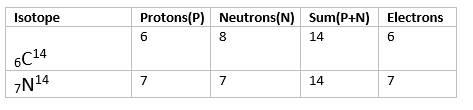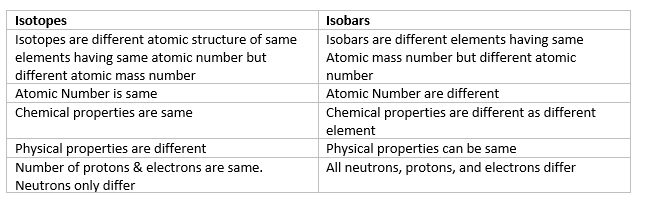# Isobars

## Isobars

• Species or atoms having same atomic mass number but different atomic number
• For example - $^{14}_{6}C$, $^{14}_{7}N$
• They have the same number of nucleons i.e sum of protons and neutrons are same but Number of protons and neutrons alone varies between them• Chemical properties depends on Atomic Number, So isobars are different chemical elements and have different chemical properties
Examples of Isobars:
(i)$^{76}_{32}Ce$,$^{76}_{34}Se$
(ii)$^{58}_{26}Fe$, $^{58}_{28}Ni$
(iii) $^{40}_{18}Ar$,$^{40}_{19}K$,$^{40}_{20}Cr$
(iv)$^{24}_{11}Na$,$^{24}_{12}Mg$
(v)$^{64}_{27}Co$,$^{64}_{28}Ni$

## Difference Between Isobars & isotopesQuestion 1
Identify the pairs which are not of isobars?
(i) $^{12}_{6}X$,$^{13}_{6}Y$
(ii)$^{35}_{17}X$,$^{37}_{17}Y$
(iii) $^{14}_{6}X$,$^{14}_{7}Y$
(iv) $^{8}_{4}X$,$^{8}_{5}Y$
Solution
Isobars have same Atomic Mass Number,So (i) and (ii) are not Isobars

Question 2
Two atoms are said to be isobars if.
(i) they have same atomic number but different mass number.
(ii) they have same number of electrons but different number of neutrons.
(iii) they have same number of neutrons but different number of electrons.
(iv) sum of the number of protons and neutrons is same but the number of protons is different.
Solution
(iv)

Question 3
Which of the following is an isobaric pair
(i) $^{13}_{6}C$,$^{13}_{7}N$
(ii)$^{13}_{6}C$,$^{14}_{7}N$
(iii)$^{14}_{7}N$,$^{15}_{8}O$
(iv) $^{13}_{7}N$,$^{15}_{8}O$
Solution
(i)

Question 4
Two nuclei which are not identical but have the same number of nucleons represent
(i) Isotopes
(ii) Isobars
(iii) Isotones
(iv) None of the above
Solution
(ii)

Question 5
The isobars are atoms with the same number of
(i) Protons
(ii) Neutrons
(iii) Protons and neutrons
(iv) Nucleons
Solution
(iv)